# Laserbeam-Figures

This instruction will show you a simple construction to use a controlled laser beam for projecting nice figures on a wall.

IntermediateFull instructions provided15 hours2,923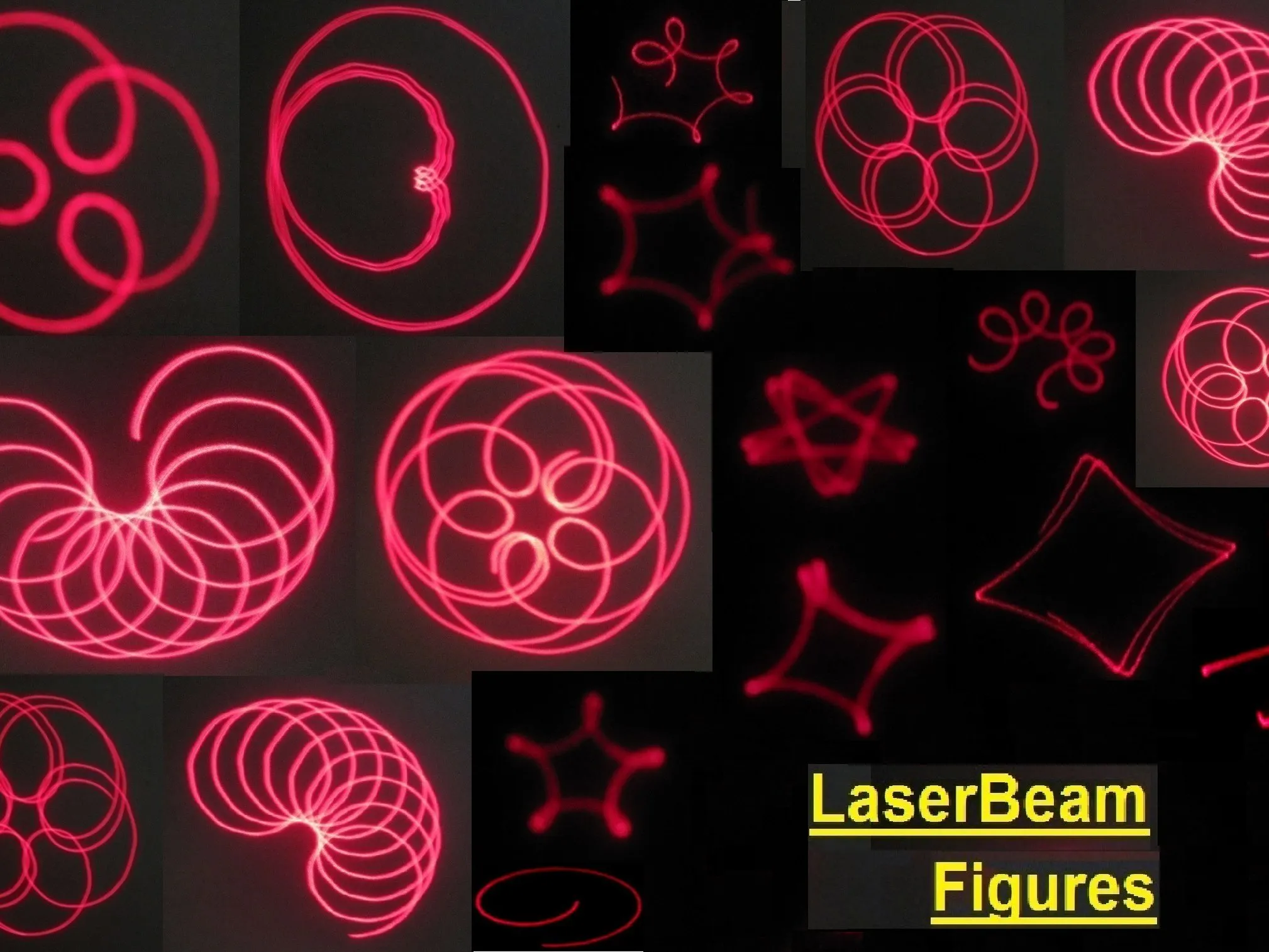## Things used in this project

### Hardware componentsArduino UNO & Genuino UNO Any other microcontroller will do, but a minimum of 3 PWM-outputs are required.
×1
 DC motor (generic) 12 - 24 Volt. from demolished printers
×3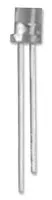Laser Diode, 2 Pins Rating 5mW. 650nm
×1

## Custom parts and enclosures

### Total construction of mounting plate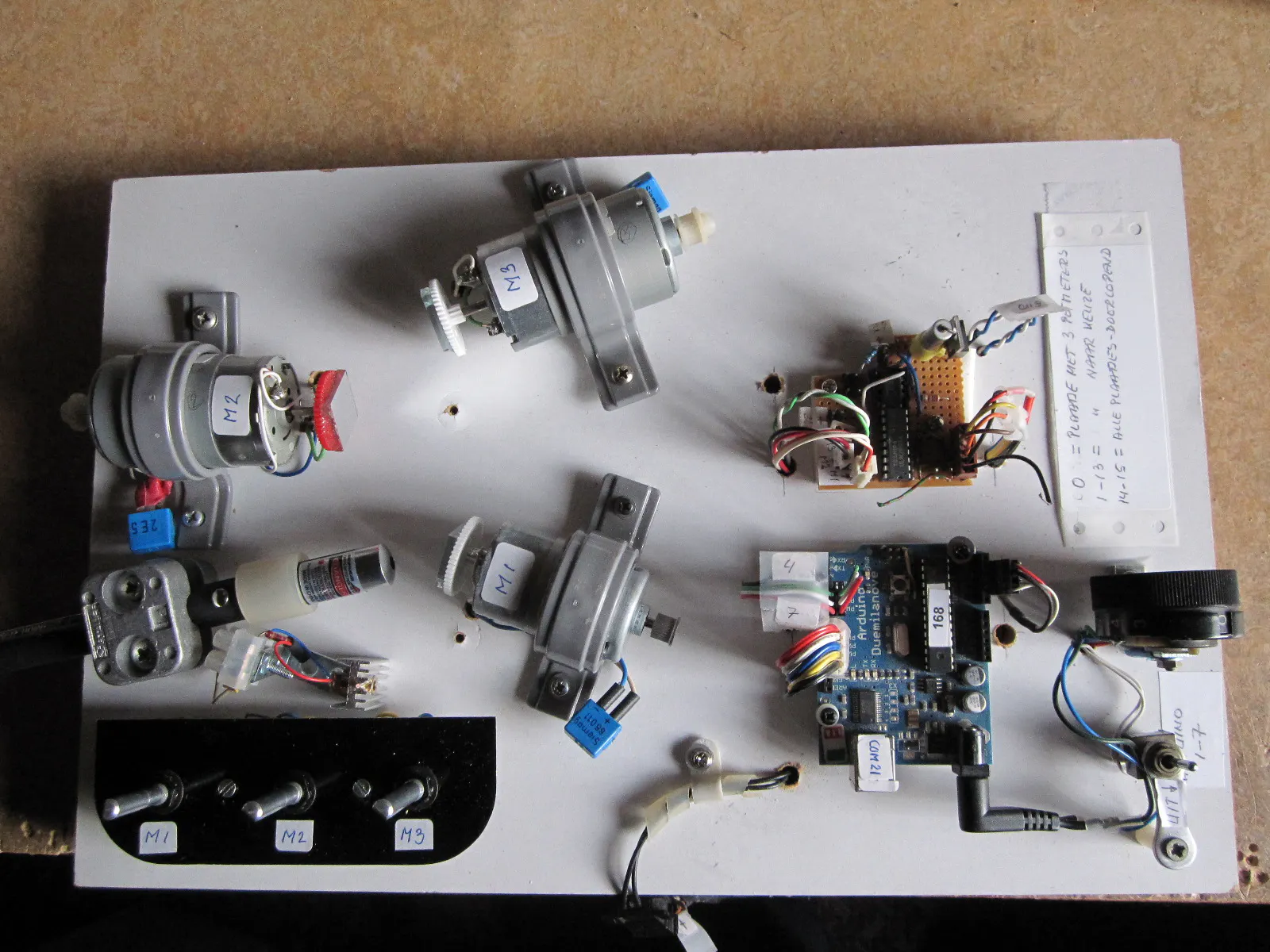### LaserBeam3.mp4

Video example figures

### IMG_0556.jpg

Example of figure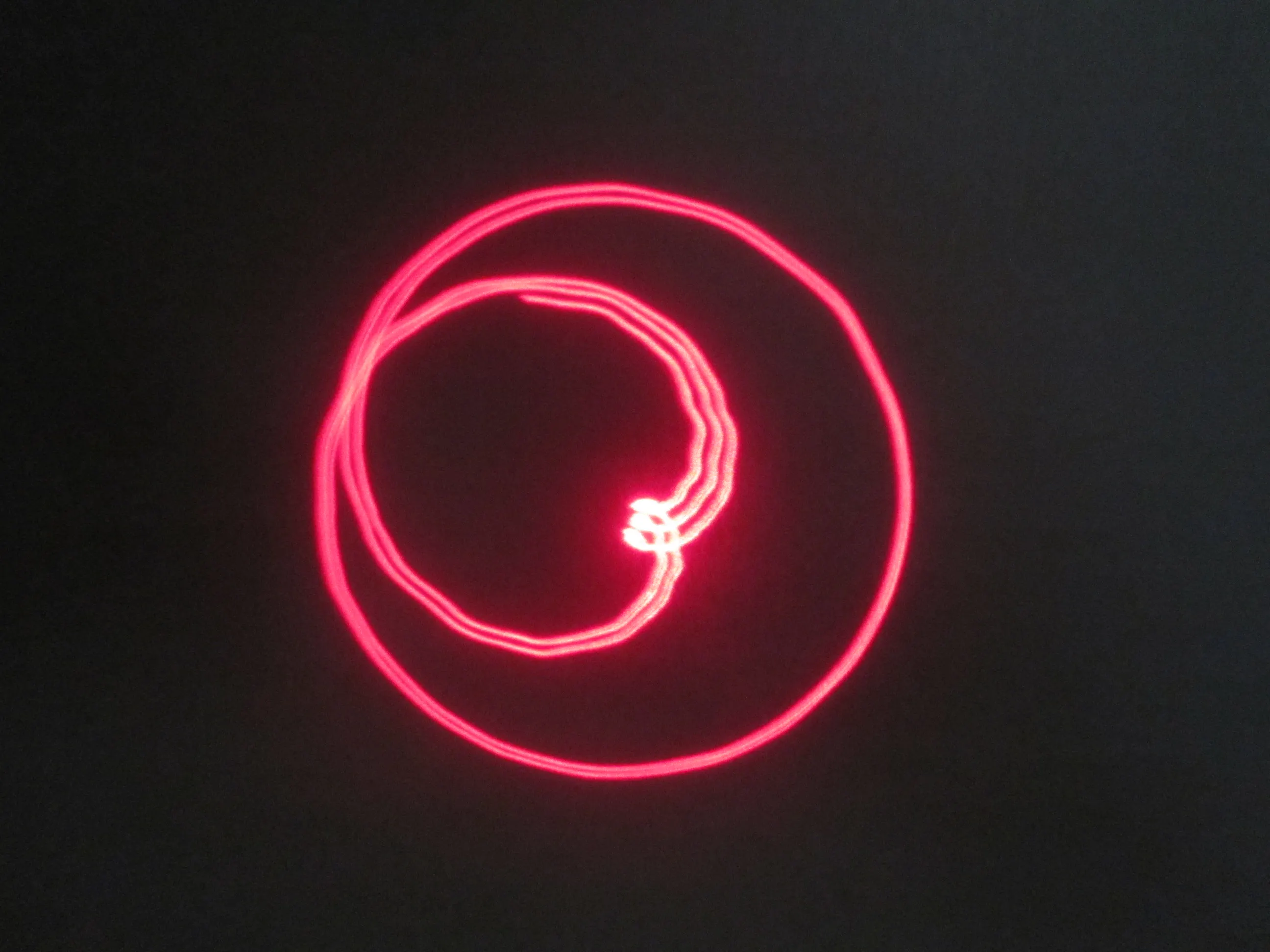### IMG_0557.jpg

Example of figure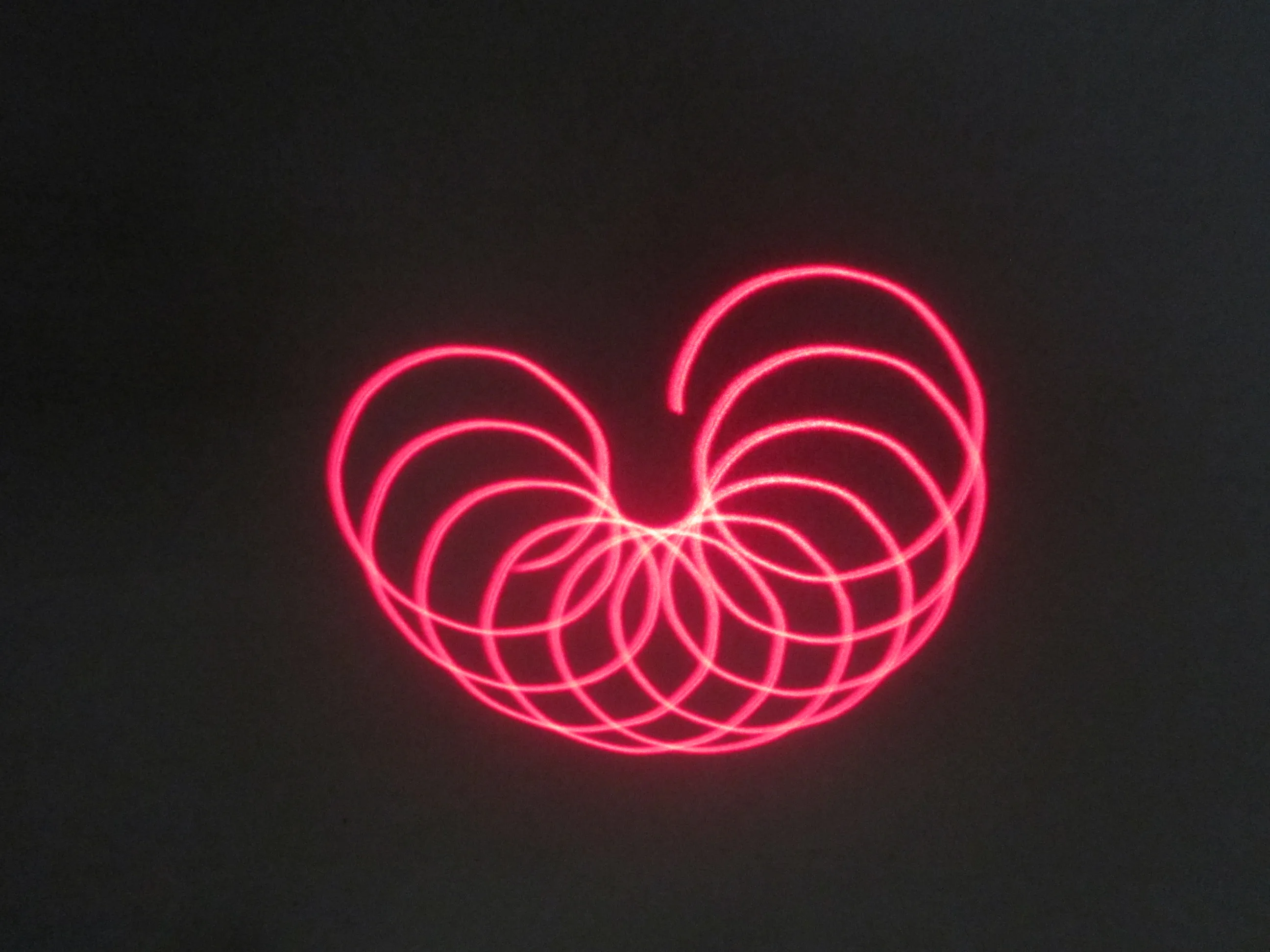### Principle Laserbeam.jpg

Principle of the system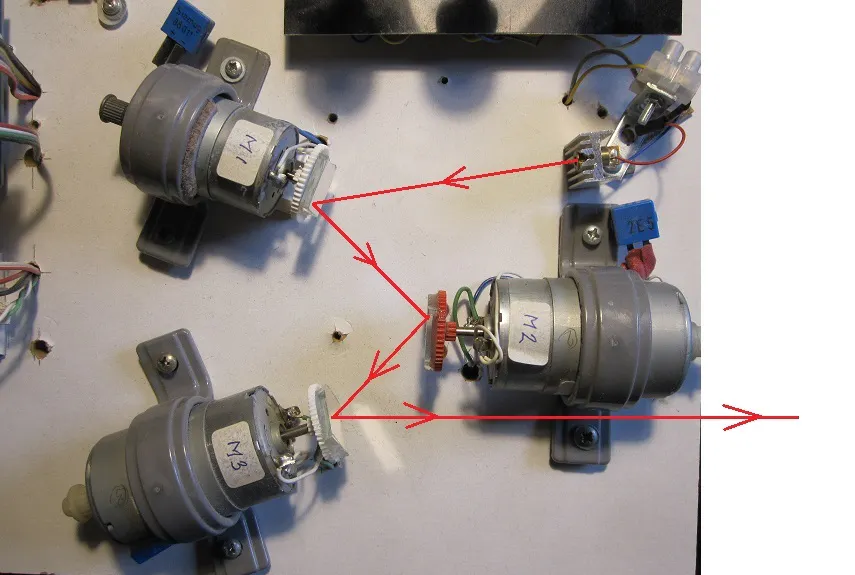### IMG_5433.jpg

Detail of mirror on motor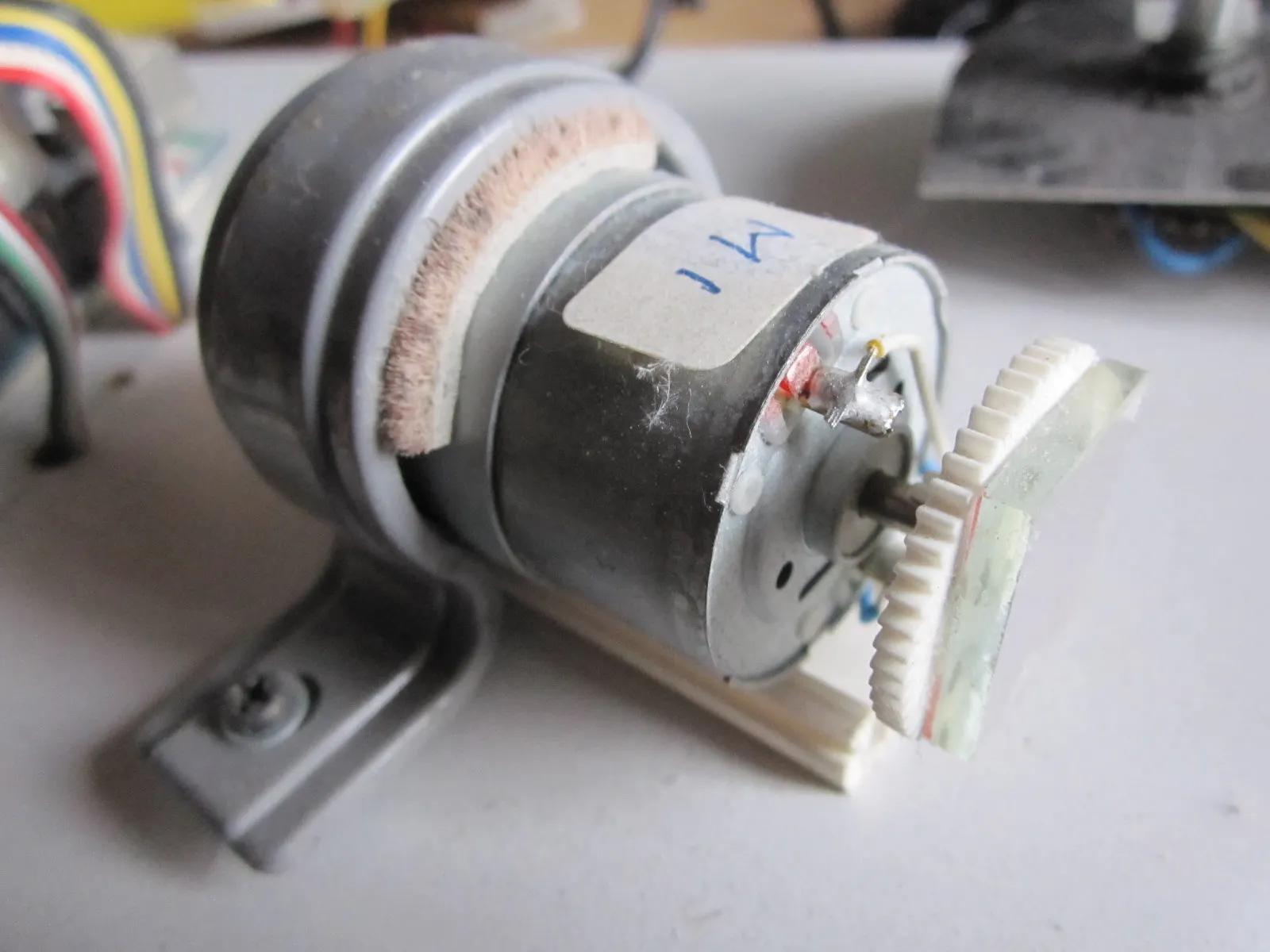### LaserBeamFigures

Some Figures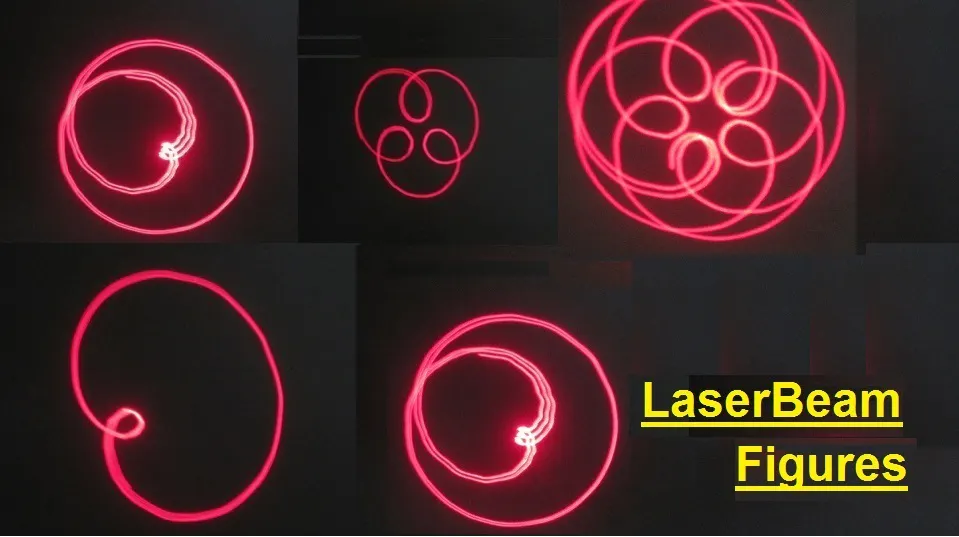## Schematics

### LaserBeam-SchemeH

Wiring Diagram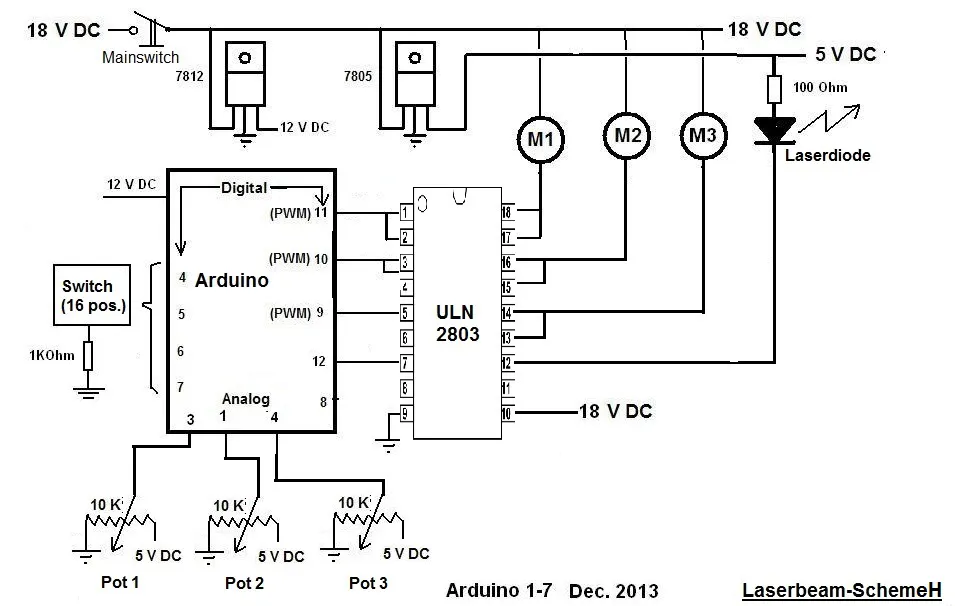## Code

### LaserBeam.ino

Arduino
```// LaserBeam with 3 Mirrors on 3 Electric motors
// Declarations :
int DataIn;
int PosSelector;    // Position of Selector (16-positions)
int Figure;
int LoopCounter;    // Determines time duration off 1 figure during "Show01()"
int Sm1, Sm2, Sm3;  // Speed motor 1 , 2 en 3 (=Output of PWM)
int Pin4 = 4;       // One of the 4 inputs for the Selector
int Pin5 = 5;       // One of the 4 inputs for the Selector
int Pin6 = 6;       // One of the 4 inputs for the Selector
int Pin7 = 7;       // One of the 4 inputs for the Selector
int Motor1 = 9;     // PWM-output for Motor
int Motor2 = 11;    // PWM-output for Motor
int Motor3 = 10;    // PWM-output for Motor
int Laser=12;       // Digital output for Laser diode

void setup()
{ Serial.begin(9600);      // Preparation Serial Communication
pinMode(Pin4, INPUT); digitalWrite (Pin4, HIGH);  // Internal Pullup
pinMode(Pin5, INPUT); digitalWrite (Pin5, HIGH);  // Internal Pullup
pinMode(Pin6, INPUT); digitalWrite (Pin6, HIGH);  // Internal Pullup
pinMode(Pin7, INPUT); digitalWrite (Pin7, HIGH);  // Internal Pullup
digitalWrite (Laser, HIGH);                       // Laser always on !!!
pinMode(Motor1, OUTPUT);
pinMode(Motor2, OUTPUT);
pinMode(Motor3, OUTPUT);
pinMode(Laser, OUTPUT);
LoopCounter=0;
Figure=999;
PosSelector=0;}

void loop()
//    Display();                 // Used only for making nice Figures
switch (PosSelector)
case 1: Figure01(); break;
case 2: Figure02(); break;
case 3: Figure03(); break;
case 4: Figure04(); break;
case 5: Figure05(); break;
case 6: Figure06(); break;
case 7: Figure07(); break;
case 8: Figure08(); break;
case 9: Figure09(); break;
case 10: Figure10(); break;
case 11: Figure11(); break;
case 12: Figure12(); break;
case 13: Figure13(); break;
default: Show01(); break;}}  // Positions 14 en 15 give all figures !!!!

void Show01()
{    LoopCounter++;
//     Display();
delay(500);    // with delay(500) avery Figure takes 5 seconden (without Display())
if (LoopCounter>0)  {if (LoopCounter<11){Figure01();}}  //
if (LoopCounter>10) {if (LoopCounter<21){Figure02();}}  //
if (LoopCounter>20) {if (LoopCounter<31){Figure03();}}  //
if (LoopCounter>30) {if (LoopCounter<41){Figure04();}}  //
if (LoopCounter>40) {if (LoopCounter<51){Figure05();}}  //
if (LoopCounter>50) {if (LoopCounter<61){Figure06();}}  //
if (LoopCounter>60) {if (LoopCounter<71){Figure07();}}  //
if (LoopCounter>70)  {if (LoopCounter<81){Figure08();}} //
if (LoopCounter>80) {if (LoopCounter<91){Figure09();}}  //
if (LoopCounter>90) {if (LoopCounter<101){Figure10();}}  //
if (LoopCounter>100) {if (LoopCounter<111){Figure11();}} //
if (LoopCounter>110) {if (LoopCounter<121){Figure12();}} //
if (LoopCounter>120) {if (LoopCounter<131){Figure13();}} //
if (LoopCounter>130) {LoopCounter=0;}}

void Figure01()  // Rotating (small) stick
{    Figure=01;
Sm1=171; Sm2=38; Sm3=253;
analogWrite(Motor1, Sm1);
analogWrite(Motor2, Sm2);
analogWrite(Motor3, Sm3);}

void Figure02()   // Propellor 4 blades
{     Figure=02;
Sm1=62; Sm2=30; Sm3=255;
analogWrite(Motor1, Sm1);
analogWrite(Motor2, Sm2);
analogWrite(Motor3, Sm3);}

void Figure03()   // Rotating dot
{    Figure=03;
Sm1=33; Sm2=0; Sm3=0;
analogWrite(Motor1, Sm1);
analogWrite(Motor2, Sm2);
analogWrite(Motor3, Sm3);}

void Figure04()   // Ovals Changing in size
{    Figure=04;
Sm1=199; Sm2=212; Sm3=202;
analogWrite(Motor1,Sm1);
analogWrite(Motor2, Sm2);
analogWrite(Motor3, Sm3);}

void Figure05()  // Rotating Ovals
{    Figure=05;
Sm1=53; Sm2=255; Sm3=255;
analogWrite(Motor1,Sm1);
analogWrite(Motor2, Sm2);
analogWrite(Motor3, Sm3);}

void Figure06()  // Flower 5 petals
{    Figure=06;
Sm1=138; Sm2=0; Sm3=57;
analogWrite(Motor1,Sm1);
analogWrite(Motor2, Sm2);
analogWrite(Motor3, Sm3);}

void Figure07()  //  Square stretched
{    Figure=07;
Sm1=48; Sm2=42; Sm3=0;
analogWrite(Motor1,Sm1);
analogWrite(Motor2, Sm2);
analogWrite(Motor3, Sm3);}

void Figure08()   // Rotating Circles
{    Figure=8;
Sm1=255; Sm2=255; Sm3=255;
analogWrite(Motor1,Sm1);
analogWrite(Motor2, Sm2);
analogWrite(Motor3, Sm3);}

void Figure09()   // Circles Changing in size
{    Figure=9;
Sm1=0; Sm2=226; Sm3=255;
analogWrite(Motor1,Sm1);
analogWrite(Motor2, Sm2);
analogWrite(Motor3, Sm3);}

void Figure10()   // Circle with internal circle
{    Figure=10;
Sm1=0; Sm2=52; Sm3=255;
analogWrite(Motor1,Sm1);
analogWrite(Motor2, Sm2);
analogWrite(Motor3, Sm3);}

void Figure11()   // Rotating Apple
{    Figure=11;
Sm1=0; Sm2=77; Sm3=255;
analogWrite(Motor1,Sm1);
analogWrite(Motor2, Sm2);
analogWrite(Motor3, Sm3);}

void Figure12()   // Rotating Circles
{    Figure=12;
Sm1=0; Sm2=42; Sm3=255;
analogWrite(Motor1,Sm1);
analogWrite(Motor2, Sm2);
analogWrite(Motor3, Sm3);}

void Figure13()   // Star
{    Figure=13;
Sm1=255; Sm2=105; Sm3=0;
analogWrite(Motor1,Sm1);
analogWrite(Motor2, Sm2);
analogWrite(Motor3, Sm3);}

void ReadDigitalInputs()  // Read 4 Switches. PosSelector has values from 0 to 15
{  PosSelector=0;

void Display()
{   Serial.print("Motor 1 : ");
Serial.print(Sm1);                  // Speed of Motor (= output of PWM)
Serial.print("   Motor 2 : ");
Serial.print(Sm2);                  // Speed of Motor (= output of PWM)
Serial.print("   Motor 3 : ");
Serial.print(Sm3);                  // Speed of Motor (= output of PWM)
Serial.print("   LoopCounter : ");
Serial.print(LoopCounter);
Serial.print("   PosSelector= ");
Serial.println(PosSelector);
//    Serial.print("   Figure : ");
//    Serial.println(Figure);
}

analogWrite(Motor1, Sm1);